# JP5097828B2 - Power converter - Google Patents

## Info

Publication number
JP5097828B2
JP5097828B2 JP2010539128A JP2010539128A JP5097828B2 JP 5097828 B2 JP5097828 B2 JP 5097828B2 JP 2010539128 A JP2010539128 A JP 2010539128A JP 2010539128 A JP2010539128 A JP 2010539128A JP 5097828 B2 JP5097828 B2 JP 5097828B2
Authority
JP
Japan
Prior art keywords
phase
voltage
output
inverter
single
Prior art date
Legal status (The legal status is an assumption and is not a legal conclusion. Google has not performed a legal analysis and makes no representation as to the accuracy of the status listed.)
Active
Application number
JP2010539128A
Other languages
Japanese (ja)
Other versions
JPWO2010058536A1 (en
Inventor

Original Assignee

Priority date (The priority date is an assumption and is not a legal conclusion. Google has not performed a legal analysis and makes no representation as to the accuracy of the date listed.)
Filing date
Publication date
Priority to JP2008294257 priority Critical
Priority to JP2008294257 priority
Application filed by 三菱電機株式会社 filed Critical 三菱電機株式会社
Priority to JP2010539128A priority patent/JP5097828B2/en
Priority to PCT/JP2009/006015 priority patent/WO2010058536A1/en
Publication of JPWO2010058536A1 publication Critical patent/JPWO2010058536A1/en
Application granted granted Critical
Publication of JP5097828B2 publication Critical patent/JP5097828B2/en
Application status is Active legal-status Critical
Anticipated expiration legal-status Critical

## Images

•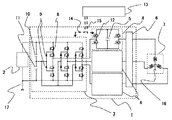•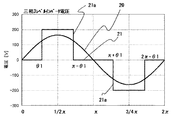•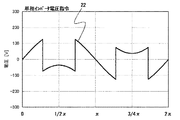•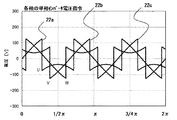•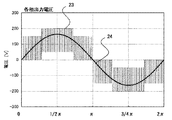•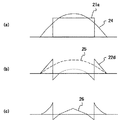•••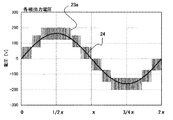••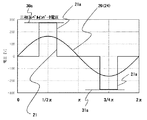•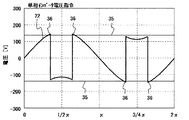••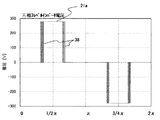••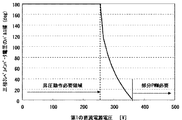•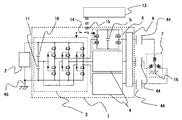•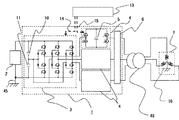## Classifications

• HELECTRICITY
• H02GENERATION; CONVERSION OR DISTRIBUTION OF ELECTRIC POWER
• H02MAPPARATUS FOR CONVERSION BETWEEN AC AND AC, BETWEEN AC AND DC, OR BETWEEN DC AND DC, AND FOR USE WITH MAINS OR SIMILAR POWER SUPPLY SYSTEMS; CONVERSION OF DC OR AC INPUT POWER INTO SURGE OUTPUT POWER; CONTROL OR REGULATION THEREOF
• H02M7/00Conversion of ac power input into dc power output; Conversion of dc power input into ac power output
• H02M7/42Conversion of dc power input into ac power output without possibility of reversal
• H02M7/44Conversion of dc power input into ac power output without possibility of reversal by static converters
• H02M7/48Conversion of dc power input into ac power output without possibility of reversal by static converters using discharge tubes with control electrode or semiconductor devices with control electrode
• H02M7/483Converters with outputs that each can have more than two voltages levels
• H02M7/49Combination of the output voltage waveforms of a plurality of converters
• HELECTRICITY
• H02GENERATION; CONVERSION OR DISTRIBUTION OF ELECTRIC POWER
• H02MAPPARATUS FOR CONVERSION BETWEEN AC AND AC, BETWEEN AC AND DC, OR BETWEEN DC AND DC, AND FOR USE WITH MAINS OR SIMILAR POWER SUPPLY SYSTEMS; CONVERSION OF DC OR AC INPUT POWER INTO SURGE OUTPUT POWER; CONTROL OR REGULATION THEREOF
• H02M7/00Conversion of ac power input into dc power output; Conversion of dc power input into ac power output
• H02M7/42Conversion of dc power input into ac power output without possibility of reversal
• H02M7/44Conversion of dc power input into ac power output without possibility of reversal by static converters
• H02M7/48Conversion of dc power input into ac power output without possibility of reversal by static converters using discharge tubes with control electrode or semiconductor devices with control electrode
• H02M7/483Converters with outputs that each can have more than two voltages levels
• H02M7/487Neutral point clamped inverters
• HELECTRICITY
• H02GENERATION; CONVERSION OR DISTRIBUTION OF ELECTRIC POWER
• H02MAPPARATUS FOR CONVERSION BETWEEN AC AND AC, BETWEEN AC AND DC, OR BETWEEN DC AND DC, AND FOR USE WITH MAINS OR SIMILAR POWER SUPPLY SYSTEMS; CONVERSION OF DC OR AC INPUT POWER INTO SURGE OUTPUT POWER; CONTROL OR REGULATION THEREOF
• H02M7/00Conversion of ac power input into dc power output; Conversion of dc power input into ac power output
• H02M7/42Conversion of dc power input into ac power output without possibility of reversal
• H02M7/44Conversion of dc power input into ac power output without possibility of reversal by static converters
• H02M7/48Conversion of dc power input into ac power output without possibility of reversal by static converters using discharge tubes with control electrode or semiconductor devices with control electrode
• H02M7/501Conversion of dc power input into ac power output without possibility of reversal by static converters using discharge tubes with control electrode or semiconductor devices with control electrode sinusoidal output voltages being obtained by the combination of several pulse-voltages having different amplitude and width
• HELECTRICITY
• H02GENERATION; CONVERSION OR DISTRIBUTION OF ELECTRIC POWER
• H02MAPPARATUS FOR CONVERSION BETWEEN AC AND AC, BETWEEN AC AND DC, OR BETWEEN DC AND DC, AND FOR USE WITH MAINS OR SIMILAR POWER SUPPLY SYSTEMS; CONVERSION OF DC OR AC INPUT POWER INTO SURGE OUTPUT POWER; CONTROL OR REGULATION THEREOF
• H02M7/00Conversion of ac power input into dc power output; Conversion of dc power input into ac power output
• H02M7/42Conversion of dc power input into ac power output without possibility of reversal
• H02M7/44Conversion of dc power input into ac power output without possibility of reversal by static converters
• H02M7/48Conversion of dc power input into ac power output without possibility of reversal by static converters using discharge tubes with control electrode or semiconductor devices with control electrode
• H02M7/483Converters with outputs that each can have more than two voltages levels
• H02M2007/4835Converters with outputs that each can have more than two voltages levels comprising a plurality of cells, each including a switchable capacitor, the capacitors having a nominal charge voltage which corresponds to a given fraction of the input voltage, the capacitors being selectively connected in series to determine the instantaneous output voltage

## Description

The present invention relates to a power conversion device that converts DC power into AC power, and in particular, power conversion that converts DC power of a DC power source having a floating capacitance such as a solar battery into AC power of three-phase output and outputs it to a load. It relates to the device.

A conventional power conversion device that converts DC power from solar cells into three-phase output AC power, and connects to a three-phase system with one phase grounded to send AC power to the system. Some conversion devices are shown below.
Connected between the output terminals of the solar cell, three sets of half-bridge inverters composed of two series switching elements, a single-phase inverter connected in series to each AC output line, and two series of voltage dividers for the voltage of the solar cell A capacitor, and each output terminal of each single-phase inverter is connected to each phase of the three-phase system. The half-bridge inverter is operated for one pulse in a half cycle, and each single-phase inverter performs PWM control so as to compensate for the shortage from the system voltage, and outputs to the system by the output sum of the half-bridge inverter and the single-phase inverter. . For this reason, the input DC voltage of the half-bridge inverter can be reduced, PWM control with a large voltage is not required, switching loss can be reduced, and the capacity of the output filter can also be reduced (see, for example, Patent Document 1).

International Publication No. 2008-102552

In the conventional power converter as described above, a three-phase two-level inverter is used as an inverter connected to the solar battery, and the DC power supply bus potential fluctuates during the inverter operation. On the other hand, the system has wiring with the V phase and midpoint grounded, and when the fluctuation of the DC power supply bus potential occurs, the floating capacitance of the DC power supply and the fluctuation of the potential cause the floating capacitance and the path of the grounding point of the system. Zero phase current is generated. This zero-phase current causes a problem that the earth leakage circuit breaker operates to stop the apparatus.

The present invention has been made to solve the above-described problems, and is a power conversion device having a small device configuration, a low cost, and a high conversion efficiency, and a floating capacitance of a DC power supply. The purpose of this is to suppress the zero-phase current passing through and prevent malfunction of the earth leakage breaker.

A power converter according to the present invention includes a three-phase three-level inverter connected between the positive and negative terminals of a first DC power source, and one or a plurality of single-phase connected in series to each phase AC output line of the three-phase three-level inverter. A phase inverter and a control device are provided, and the sum of the output voltage of the three-phase three-level inverter and the output voltage of each single-phase inverter is output to a load via a smoothing filter. A second DC power supply, which is a DC input power supply for the single-phase inverter, is constituted by a DC capacitor, and the voltage of the second DC power supply is smaller than the one-level voltage of the three-phase three-level inverter. Further, the control device is configured such that each phase of the three-phase three-level inverter outputs one pulse voltage as a main voltage pulse with respect to a half cycle of each phase output voltage to the load. And the pulse width of the main voltage pulse output from the three-phase three-level inverter is controlled so that the half-cycle or one-cycle output power balance of each single-phase inverter becomes zero. The phase inverter is PWM-controlled, and each phase output voltage to the load is different from each other by 2π / 3 with reference to the point having zero or constant DC potential from the reference potential of the first DC power supply. be one that controlled to be a sine wave having the same peak value in the phase, when the voltage value of the second DC power supply of the individual single-phase inverters is larger than the reference value of the corresponding phase the main voltage pulse The pulse width shorter, when the voltage value of the second DC power supply is smaller than the reference value is to lengthen the pulse width of the main voltage pulse corresponding phase.
The power converter according to the present invention is connected in series with one or more three-phase three-level inverters connected between the positive and negative terminals of the first DC power source and each phase AC output line of the three-phase three-level inverter. A single-phase inverter and a control device, and outputs the sum of the output voltage of the three-phase three-level inverter and the output voltage of each single-phase inverter to a load via a smoothing filter. A second DC power supply, which is a DC input power supply for the single-phase inverter, is constituted by a DC capacitor, and the voltage of the second DC power supply is smaller than the one-level voltage of the three-phase three-level inverter. In addition, the control device may be configured so that each phase of the three-phase three-level inverter has one main voltage pulse for the half cycle of each phase output voltage to the load, the vicinity of the rising portion of the main voltage pulse, and the rising edge. The three-phase three-level inverter is controlled so as to output a multi-pulse by PWM control in the vicinity of the downstream portion, and the half-cycle or one-cycle output power balance of each single-phase inverter is zero. The pulse width of the main voltage pulse output from the three-phase three-level inverter and the voltage output period by the PWM control are controlled, and each single-phase inverter is PWM-controlled so that each phase output voltage to the load is Controls each phase to be a sine wave having the same peak value at a phase that is 2π / 3 different from the reference potential of one DC power supply with zero or a constant DC potential as a reference. When the voltage value of the second DC power source of each single-phase inverter is larger than a reference value, the voltage output period of the three-phase three-level inverter of the corresponding phase is shortened, and the second When the voltage value of the DC power source is smaller than the reference value, the voltage output period of the three-phase three-level inverter of the corresponding phase is lengthened.

The power conversion device according to the present invention is capable of controlling the output voltage to be a sine wave having the same peak value with a phase that is 2π / 3 different from each other with a predetermined DC potential point of the first DC power supply as a reference. And the fluctuation of the bus potential of the first DC power supply can be eliminated. For this reason, it can be set as the apparatus structure which does not have an alternating current component between the neutral potential of a three-phase output, and one electric potential of a 1st DC power supply, and the zero phase which flows through the floating electrostatic capacitance of a 1st DC power supply Current can be suppressed, and malfunction of the earth leakage breaker can be prevented.
Further, since the three-phase three-level inverter and the single-phase inverter are combined and output, the input DC voltage of the three-phase three-level inverter may be low, and there is no need for PWM control with a large voltage. For this reason, it becomes a power converter device with high conversion efficiency with a small device configuration and low cost.

It is a figure which shows the structure of the power converter device by Embodiment 1 of this invention. It is a voltage waveform diagram explaining the operation | movement of the three-phase inverter circuit by Embodiment 1 of this invention. It is a voltage waveform diagram explaining the operation | movement of the three-phase inverter circuit by Embodiment 1 of this invention. It is a voltage waveform diagram explaining the operation | movement of the three-phase inverter circuit by Embodiment 1 of this invention. It is a voltage waveform diagram explaining the operation | movement of the three-phase inverter circuit by Embodiment 1 of this invention. It is a wave form diagram explaining the comparative example of the operation | movement of the three-phase inverter circuit by Embodiment 2 of this invention. It is a wave form diagram explaining operation | movement of the three-phase inverter circuit by Embodiment 2 of this invention. It is a figure which shows the structure of the power converter device by Embodiment 4 of this invention. It is a voltage waveform diagram explaining operation | movement of the three-phase inverter circuit by Embodiment 4 of this invention. It is a figure which shows the structure of the power converter device by Embodiment 5 of this invention. It is a voltage waveform diagram explaining the comparative example of the operation | movement of the three-phase inverter circuit by Embodiment 5 of this invention. It is a voltage waveform diagram explaining the comparative example of the operation | movement of the three-phase inverter circuit by Embodiment 5 of this invention. It is a voltage waveform diagram explaining operation | movement of the three-phase inverter circuit by Embodiment 5 of this invention. It is a voltage waveform diagram explaining operation | movement of the three-phase inverter circuit by Embodiment 5 of this invention. It is a figure which shows the structure of the power converter device by Embodiment 6 of this invention. It is a figure explaining operation | movement of the three-phase inverter circuit by Embodiment 6 of this invention. It is a figure which shows the structure of the power converter device by Embodiment 7 of this invention. It is a figure which shows the structure of the power converter device by Embodiment 8 of this invention.

Embodiment 1 FIG.
Hereinafter, a power converter according to Embodiment 1 of the present invention will be described with reference to the drawings.
1 is a diagram showing a configuration of a power conversion device according to Embodiment 1 of the present invention. The power conversion device includes a three-phase inverter circuit 1 and an output control device 13 which are main circuits. The three-phase inverter circuit 1 converts the DC power from the first DC power source 2 into three-phase AC power and outputs it to the load 7. The first DC power source 2 It is a direct current power source such as a solar battery having a capacity 17. The load 7 is grounded at a load ground point 16.
The three-phase inverter circuit 1 includes a three-phase three-level inverter 3 that uses the voltage of the first DC power supply 2 as a bus voltage, and a single-phase inverter 4 that is connected in series to each phase AC output line of the three-phase three-level inverter 3. And a three-phase smoothing filter 6 that is connected to the subsequent stage of the single-phase inverter 4 and includes a reactor and a capacitor (not shown).

Each phase of the three-phase three-level inverter 3 is composed of four semiconductor switching elements 8 made of IGBT or the like each having a diode connected in antiparallel, and two clamp diodes 9. The three-phase three-level inverter 3 includes a first series capacitor 10 and a second series capacitor 11 as two series capacitors that divide the first DC power supply 2, and includes the first series capacitor 10 and the second series capacitor 10. The connection point with the series capacitor 11 is connected to the connection point between the two clamp diodes 9 of each phase. That is, the connection point between the first series capacitor 10 and the second series capacitor 11 is a neutral point that is a potential point that divides the voltage of the first DC power supply 2 into two, and constitutes the upper and lower arms of each phase. The connection point between the two semiconductor switching elements 8 is clamped at the neutral point potential.

Each single-phase inverter 4 includes a full-bridge inverter 12 composed of four semiconductor switching elements, and a DC capacitor 5 as a second DC power source that holds a voltage. The output voltage of the single-phase inverter 4 of each phase is superimposed on the output voltage of each phase of the three-phase three-level inverter 3, and the voltage sum of the output voltage of the three-phase three-level inverter 3 and the output voltage of each single-phase inverter 4 Is output to the load 7 through the smoothing filter 6.
The voltage of the DC capacitor 5 of each single-phase inverter 4 is set to be smaller than 1/2 of the voltage of the first DC power supply 2 (or the voltage of the first and second series capacitors 10 and 11). Yes. That is, the voltage of the DC capacitor 5 is smaller than the one-level voltage of the three-phase three-level inverter 3. In FIG. 1, for convenience, among the three single-phase inverters 4, only one phase of the circuit configuration is illustrated and the other phases are omitted.
The three-phase three-level inverter 3 and each single-phase inverter 4 are driven by a three-phase three-level inverter control signal 14 and a single-phase inverter control signal 15 output from an output control device 13 that can be operated by a CPU, DSP, FPGA, or the like. Be controlled.

The operation of the three-phase inverter circuit 1 configured as described above will be described below based on the voltage waveforms shown in FIGS. The waveform potential is a potential with reference to a neutral point, which is a connection point between the first series capacitor 10 and the second series capacitor 11.
FIG. 2 shows a voltage waveform (three-phase three-level inverter) output by one phase output from the three-phase inverter circuit 1, for example, a phase voltage command 20 that is a U-phase voltage command, and a U-phase of the three-phase three-level inverter 3. Voltage 21). Note that the phase voltage command 20 is a sine wave in which each phase is different by 2π / 3 and has the same peak value.
The DC voltage output from the first DC power supply 2 is charged in the series body of the first series capacitor 10 and the second series capacitor 11. The voltages of the first DC power supply 2, the first series capacitor 10, and the second series capacitor 11 are detected, and each detected voltage value is transmitted to the output control device 13.

By the three-phase three-level inverter control signal 14 from the output control device 13, each phase of the three-phase three-level inverter 3 takes the DC voltage of the first series capacitor 10 and the second series capacitor 11 as input voltage, The peak value corresponding to each voltage value of the series capacitor 10 and the voltage value of the second series capacitor 11 (or a voltage value that is half the voltage of the first DC power supply 2), in this case, a voltage of 200V Pulses are output at a rate of one pulse per half cycle with respect to the phase voltage command 20. Hereinafter, the voltage pulse of one pulse in this half cycle is referred to as a main voltage pulse 21a. Here, in one cycle of the phase voltage command 20, one pulse of the main voltage pulse 21a is output on the positive side and one pulse on the negative side of the phase voltage command 20. The main voltage pulse 21a is output so that the power balance of the half cycle (or one cycle) of the single-phase inverter 4 becomes 0. Details of this control will be described later.

FIG. 3 shows an output voltage command (single-phase inverter voltage command 22) of the single-phase inverter 4. This single-phase inverter voltage command 22 is obtained by subtracting the three-phase three-level inverter voltage 21 of each phase from the phase voltage command 20 of the three-phase inverter circuit 1. Each single-phase inverter 4 has a difference between a phase voltage command 20 required for the three-phase inverter circuit 1 and an output voltage of each phase of the three-phase three-level inverter 3 by a single-phase inverter control signal 15 from the output control device 13. The output is controlled by high frequency PWM so as to compensate. In addition, each single-phase inverter 4 is controlled so that each phase output current to the load 7 becomes a sine wave in this PWM control.
FIG. 4 shows a single-phase inverter voltage command of the single-phase inverter 4 for each phase, 22a is a U-phase single-phase inverter voltage command, 22b is a V-phase single-phase inverter voltage command, and 22c is a W-phase single-phase inverter voltage command. is there. In this case, the waveform peak value is ± 125 V, and the bus voltage of each single-phase inverter 4 is required to be 125 V or more in order to output the voltage command voltage.

The output voltage of the single-phase inverter 4 of each phase is superimposed on the output voltage of each phase of the three-phase three-level inverter 3, and the voltage sum of the output voltage of the three-phase three-level inverter 3 and the output voltage of each single-phase inverter 4 Is output to the load 7 through the smoothing filter 6. FIG. 5 shows each phase output voltage of the three-phase inverter circuit 1 which is the voltage sum of the output voltage of the three-phase three-level inverter 3 and the output voltage of each single-phase inverter 4. Reference numeral 23 denotes each phase output voltage, and 24 denotes an average voltage waveform of each phase output voltage 23.
Each phase output voltage waveform 24 to the load 7 is the same voltage waveform as the phase voltage command 20 of each phase, that is, a neutral point that is a connection point between the first series capacitor 10 and the second series capacitor 11. Each phase is a sine wave having the same peak value, with each phase being different by 2π / 3 with respect to the potential.

Next, the output control of the main voltage pulse 21a of the three-phase three-level inverter 3 and the power balance of the single-phase inverter 4 will be described below with reference to FIG.
As described above, the main voltage pulse 21a is output so that the half-cycle or single-cycle power balance of the single-phase inverter 4 becomes zero. Since the single-phase inverter 4 outputs so as to compensate for the difference between the phase voltage command 20 and the output voltage of each phase of the three-phase three-level inverter 3, the three-phase three-level inverter 3 outputs the power output by the phase voltage command 20 It is sufficient to output the same power as the main voltage pulse 21a.
When controlling the phase of the output current to match the phase of the output voltage (power factor 1 operation), the peak voltage of the phase voltage command 20 is Vp, and the DC voltage (here, the first voltage input to the three-phase inverter circuit 1) When Ed is 1/2 of the voltage of the DC power supply 2 or the sum of the voltage of the first series capacitor 10 and the voltage of the second series capacitor 11, Vp can be expressed by the following equation (1). However, θ1 (0 <θ1 <π / 2) is a phase in which the main voltage pulse 21a rises.

From the above equation (1), the phase θ1 at which the main voltage pulse 21a rises is represented by the following equation (2).

A voltage pulse having a pulse width of (π−2θ1) rising at the phase (nπ + θ1) calculated in this way becomes the main voltage pulse 21a. The output control device 13 performs the above calculation, sends a three-phase three-level inverter control signal 14 based on the calculation result to the three-phase three-level inverter 3, and controls the output of the three-phase three-level inverter 3. In the above calculation, the phase θ1 at which the main voltage pulse 21a rises is calculated. Determining this phase θ1 is the same as determining the pulse width (π−2θ1).

In this embodiment, a three-phase three-level inverter 3 is used as an inverter connected to the first DC power supply 2, and the entire three-phase inverter circuit 1 is connected to the first series capacitor 10 and the second series capacitor 11. With reference to the potential of the neutral point, which is a point, each phase was controlled to be a sine wave having the same peak value with a phase different by 2π / 3. In such a configuration, the neutral point potential becomes stable, the bus potential of the first DC power supply 2 does not fluctuate, the output voltage control that can obtain a desired voltage waveform can be realized with high accuracy, and the three-phase output voltage total Becomes 0. Therefore, a zero-phase current that flows through the floating capacitance 17 of the first DC power supply 2 without having an AC component between the neutral potential of the three-phase output and one potential of the first DC power supply 2. Can be suppressed. The zero-phase current is normally detected as a leakage current in the leakage breaker arranged in the preceding stage of the load 7, but the zero-phase current can be suppressed, so that the malfunction of the leakage breaker can be prevented and the leakage current is reduced. Therefore, the power conversion efficiency of the three-phase inverter circuit 1 is improved.

Further, since the three-phase inverter circuit 1 outputs a voltage that is the sum of the output voltage of the three-phase three-level inverter 3 and the output voltage of each single-phase inverter 4, the three-phase inverter circuit 1 is the first DC input voltage of the three-phase inverter circuit 1. A voltage higher than the voltage of the DC power source 2 can be output. Further, each phase of the three-phase three-level inverter 3 is operated by one pulse in a half cycle, so that almost no switching loss occurs. Since the DC voltage of the single-phase inverter 4 that is PWM controlled at a high frequency is selected to be a relatively small value, the switching loss due to PWM control is small, and the capacity of the smoothing filter 6 may be small. For this reason, the three-phase inverter circuit 1 has a small, low-cost and high conversion efficiency device configuration.

In this embodiment, since the three-phase inverter circuit 1 is controlled so that the half-cycle or one-cycle power balance of each single-phase inverter 4 becomes 0, the DC capacitor 5 of each single-phase inverter 4 is externally connected. It is possible to make a simple configuration without a DC power source for transmitting and receiving power.

Embodiment 2. FIG.
In the first embodiment, the three-phase, three-level inverter 3 has the pulse width (or rising phase) of the main voltage pulse 21a so that the power equivalent to the power output by the phase voltage command 20 is output by the main voltage pulse 21a. However, the pulse width can be determined by other methods. In this embodiment, the half-cycle or one-cycle power integrated value of the single-phase inverter 4 is calculated, and the pulse width of the main voltage pulse 21a is obtained so that the power integrated value becomes zero.
The relationship between the pulse width of the main voltage pulse 21a of the three-phase three-level inverter 3 and the output power of the single-phase inverter 4 will be described below with reference to FIGS. FIG. 6 is a comparative example in which the half-cycle integrated power value of the single-phase inverter 4 is positive. FIG. 7 shows that the main voltage pulse 21a has a wider pulse width than the case of FIG. The case where the electric power integration value of a period is set to 0 is shown. For convenience, only a half-cycle waveform is shown.

As shown in FIGS. 6A and 7A, for each phase output voltage waveform 24 of the three-phase inverter circuit 1, the three-phase three-level inverter 3 generates one main voltage pulse 21a in a half cycle. Output.
Then, as shown in FIGS. 6 (b) and 7 (b), a single phase is obtained so that a voltage waveform of the difference between the main voltage pulse 21a of the three-phase three-level inverter 3 and each phase output voltage waveform 24 is obtained. The inverter 4 outputs an average voltage 22d by PWM control. For example, when the three-phase three-level inverter 3 is a solar power conditioner, the output current to the load 7 is often a power factor of 1. When the power factor is 1, the current waveform of the output current 25 is a sine wave having the same phase as each phase output voltage waveform 24.

The output power 26 of the single-phase inverter 4 that is the product of the output voltage 22d and the output current 25 has the waveforms shown in FIGS. 6 (c) and 7 (c). In FIG. 6C, since the integrated value of the half cycle of the output power 26 of the single phase inverter 4 is positive, the DC capacitor 5 of the single phase inverter 4 requires an external power source. In FIG.7 (c), the pulse width of the main voltage pulse 21a is expanded, In this case, the negative output power 26 of the single phase inverter 4 increases, and the half-cycle integrated power value becomes zero.

In this embodiment, the pulse width of the main voltage pulse 21a is determined so that the half-cycle or single-cycle power integrated value of the single-phase inverter 4 becomes zero. Since the power balance of each single-phase inverter 4 is controlled so that the half-cycle or one-cycle power balance becomes zero, the DC capacitor 5 of each single-phase inverter 4 can have a simple configuration that does not have a DC power supply for transmitting and receiving power from the outside. .

Embodiment 3 FIG.
In the first and second embodiments, the pulse width of the main voltage pulse 21a is determined so that the half-cycle or one-cycle power balance of the single-phase inverter 4 is zero. In this embodiment, a fine adjustment of the pulse width of the main voltage pulse 21a is shown. In this case, each voltage detector 32 (see FIG. 10) is provided to measure the voltage of each DC capacitor 5 of each single-phase inverter 4.
First, similarly to the first and second embodiments, the three-phase inverter circuit 1 is output by determining the pulse width of the main voltage pulse 21a so that the half-cycle or single-cycle power balance of the single-phase inverter 4 becomes zero. Control. In this output control, when the balance of power balance of the single-phase inverter 4 is lost due to some cause, such as a sudden change in the input DC voltage or a sudden change in the load 7, the voltage of the DC capacitor 5 of the single-phase inverter 4 changes.

Each voltage detector 32 detects the voltage of each DC capacitor 5 of each single-phase inverter 4, and the detected voltage value of each DC capacitor 5 is transmitted to the output control device 13. In the output control device 13, when the voltage value of the DC capacitor 5 is larger than a preset reference value, the pulse width of the main voltage pulse 21a of the corresponding phase is shortened, and when the voltage value is larger than the reference value, the pulse width is increased. The three-phase three-level inverter 3 is controlled so that the voltage value of the DC capacitor 5 becomes longer and approaches the reference value.

As described in the second embodiment, the half-cycle or one-cycle power integrated value of the single-phase inverter 4 increases when the pulse width of the main voltage pulse 21a is shortened and when the pulse width of the main voltage pulse 21a is increased. Reduce. For this reason, when the voltage value of the DC capacitor 5 is larger than the reference value, the pulse width of the main voltage pulse 21a of the corresponding phase is shortened, thereby increasing the power integrated value of the single-phase inverter 4 and Reduce the voltage. Further, when the voltage value of the DC capacitor 5 is smaller than the reference value, the integrated value of the main voltage pulse 21a of the corresponding phase is lengthened, thereby reducing the integrated power value of the single-phase inverter 4 and the voltage of the DC capacitor 5 Increase

In this way, feedback control is performed so that the voltage of the DC capacitor 5 of each single-phase inverter 4 becomes the reference value by adjusting the pulse width of the main voltage pulse 21a output from the three-phase three-level inverter 3. Thereby, the half-cycle or one-cycle power balance of each single-phase inverter 4 can be reliably controlled to zero. For this reason, shortage of output voltage of the single-phase inverter 4, overcharge of the DC capacitor 5, and insulation breakdown of the single-phase inverter 4 due to overcharge can be prevented, and the three-phase inverter circuit 1 with stable output can be obtained. Can do.

Embodiment 4 FIG.
Next, a power converter according to Embodiment 4 of the present invention will be described with reference to the drawings.
FIG. 8 is a diagram showing a configuration of a power conversion device according to Embodiment 4 of the present invention. In the three-phase inverter circuit 1 of this embodiment, a plurality of (two in this case) single-phase inverters 4, 4 a are connected in series to each phase AC output line of the three-phase three-level inverter 3. The two single-phase inverters 4 and 4a in each phase have the same configuration, and for convenience, only one single-phase inverter 4 is illustrated as a circuit configuration, and the others are omitted.
The three-phase three-level inverter 3 and each single-phase inverter 4, 4 a are a three-phase three-level inverter control signal 14 output from an output control device 13 a that can be operated by a CPU, DSP, FPGA, etc., and single-phase inverter control. The drive is controlled by signals 15 and 15a.
Other configurations are the same as those shown in FIG. 1 of the first embodiment.

Next, the operation will be described.
Each phase of the three-phase three-level inverter 3 outputs the main voltage pulse 21a at a rate of one pulse per half cycle with respect to the phase voltage command 20 as in the first embodiment. The main voltage pulse 21a is output with a pulse width determined so that the power balance of the half cycle (or one cycle) of the single-phase inverter 4 becomes zero.
Each single-phase inverter 4, 4 a is output under high-frequency PWM control so as to compensate for the difference between the phase voltage command 20 required for the three-phase inverter circuit 1 and the output voltage of each phase of the three-phase three-level inverter 3. In this case, the difference between the phase voltage command 20 and the output voltage of each phase of the three-phase three-level inverter 3 is compensated by the sum of the output voltages of the two single-phase inverters 4 and 4a in each phase. Each single-phase inverter 4, 4 a is controlled so that the output current becomes a sine wave in this PWM control.

The output voltage of the two single-phase inverters 4 and 4a in each phase is superimposed on the output voltage of each phase of the three-phase three-level inverter 3, and the output voltage of the three-phase three-level inverter 3 and each single-phase inverter 4 and 4a. Is output to the load 7 through the smoothing filter 6.
FIG. 9 shows each phase output voltage of the three-phase inverter circuit 1 which is the voltage sum of the output voltage of the three-phase three-level inverter 3 and the output voltages of the two single-phase inverters 4 and 4a. Reference numeral 23a denotes each phase output voltage, and 24 denotes an average voltage waveform of each phase output voltage 23. In this case, the two single-phase inverters 4 and 4a connected in series output by shifting the switching timing by shifting the phase of the carrier wave by 180 °, for example.
Similarly to the first embodiment, each phase output voltage waveform 24 to the load 7 has the same voltage waveform as the phase voltage command 20 of each phase, that is, the first series capacitor 10 and the second series capacitor 11. Each phase becomes a sine wave having the same peak value with a phase that is different by 2π / 3 with respect to the potential of the neutral point that is a connection point with the reference point.

Also in this embodiment, as in the first embodiment, the neutral point potential is stable, the bus potential of the first DC power supply 2 does not fluctuate, and the output voltage control for obtaining a desired voltage waveform is highly accurate. The total output voltage of the three phases becomes zero. Therefore, a zero-phase current that flows through the floating capacitance 17 of the first DC power supply 2 without having an AC component between the neutral potential of the three-phase output and one potential of the first DC power supply 2. Can be suppressed.
In addition, since a plurality of (two in this case) single-phase inverters 4 and 4a are connected in series to each phase AC output line of the three-phase three-level inverter 3, the voltage output from each single-phase inverter 4 and 4a is reduced. And switching loss is reduced. Further, when the number of single-phase inverters 4 and 4a is increased, the frequency of the carrier wave may be lowered, and the switching loss is further reduced.

Of the two single-phase inverters 4 and 4a connected in series, one single-phase inverter 4 has an output of a few pulses or less in a half cycle, and only the other single-phase inverter 4a is output by high-frequency PWM control. Also good. At this time, the DC voltage of the single-phase inverter 4a to be PWM controlled may be lower than the DC voltage of the single-phase inverter 4.

Embodiment 5 FIG.
Next, a power converter according to Embodiment 5 of the present invention will be described with reference to the drawings.
FIG. 10 is a diagram showing a configuration of a power conversion device according to Embodiment 5 of the present invention. In this embodiment, the voltage detector 30 provided in parallel to measure the voltage of the first series capacitor 10 and the voltage detection provided in parallel to measure the voltage of the second series capacitor 11 are measured. And a voltage detector 32 provided for measuring the voltage of each DC capacitor 5 of each single-phase inverter 4. The voltage values 30a to 32a detected by these voltage detectors 30 to 32 are transmitted to the output control device 13, and the output control device 13 is based on the detected voltage values 30a to 32a. 3 and each single-phase inverter 4 are controlled.
Other configurations are the same as those shown in FIG. 1 of the first embodiment.

In the first embodiment, the three-phase three-level inverter 3 outputs only one main voltage pulse 21a in a half cycle, but in the fifth embodiment, the three-phase three-level inverter 3 operates differently. do. FIG. 11 to FIG. 14 are voltage waveforms for explaining the operation of the three-phase inverter circuit 1 according to the fifth embodiment. The waveform potential is a potential with reference to a neutral point, which is a connection point between the first series capacitor 10 and the second series capacitor 11. 11 and 12 show a comparative example, and FIGS. 13 and 14 show voltage waveforms of the three-phase inverter circuit 1 according to the fifth embodiment.

If the operation is the same as in the first embodiment, the phase voltage command 20 (each phase output voltage waveform 24) of the three-phase inverter circuit 1 and the voltage waveform output by each phase of the three-phase three-level inverter 3 (three The phase 3 level inverter voltage 21) is shown in FIG. The voltage of the main voltage pulse 21a output to the positive side of the phase voltage command 20 is the voltage value 30a of the first series capacitor 10, and the voltage of the main voltage pulse 21a output to the negative side of the phase voltage command 20 ( (Absolute value) is the voltage value 31 a of the second series capacitor 11. In this case, the voltage of the first DC power supply 2 is higher than the normal case shown in FIG. 2, and the voltage values 30a and 31a of the first and second series capacitors 10 and 11 are higher. Yes.
As described above, the pulse width of the main voltage pulse 21a is determined so that the half-cycle or one-cycle power balance of the single-phase inverter 4 becomes 0. Therefore, the DC input voltage of the three-phase three-level inverter 3 is large. Then, as shown in the figure, the pulse width becomes shorter.

When each phase of the three-phase three-level inverter 3 outputs the three-phase three-level inverter voltage 21 as shown in FIG. 11, the output voltage command (single-phase inverter voltage command 22) of the single-phase inverter 4 is shown in FIG. Show. The single-phase inverter voltage command 22 is obtained by subtracting the three-phase three-level inverter voltage 21 from the phase voltage command 20 of the three-phase inverter circuit 1.
The single-phase inverter 4 needs to generate a difference voltage between the phase voltage command 20 of the three-phase inverter circuit 1 and the three-phase three-level inverter voltage 21, and this difference voltage is generated by the first and second series capacitors 10. , 11 increases and the pulse width of the main voltage pulse 21a becomes shorter. For this reason, as shown in the figure, a voltage 36 exceeding the output voltage limit value 35 of the single-phase inverter 4 is required in the vicinity of the rising portion and the falling portion of the main voltage pulse 21a. Note that the magnitude of the output voltage limit value 35 is the voltage value 32 a of the DC capacitor 5 of the single-phase inverter 4.

FIG. 13 is a diagram showing an output voltage command (single-phase inverter voltage command 37) of the single-phase inverter 4 according to this embodiment, and FIG. 14 shows each phase of the three-phase three-level inverter 3 according to this embodiment. The output voltage waveform (three-phase three-level inverter voltage) is shown.
Since the single-phase inverter 4 cannot output the voltage 36 exceeding the output voltage limit value 35 as shown in FIG. 12, during the period when the voltage 36 exceeding the output voltage limit value 35 is required, as shown in FIG. The shortage of the output voltage of the single-phase inverter 4 is borne by the three-phase three-level inverter 3. That is, the three-phase three-level inverter 3 outputs a partial PWM voltage 38, which is a voltage by PWM control, in the vicinity of the rising portion and the falling portion of the main voltage pulse 21a, and the output voltage of the single-phase inverter 4 Then output the insufficient voltage.

The partial PWM voltage 38 is output according to the voltage values 30 a and 31 a of the first and second series capacitors 10 and 11 and the voltage value 32 a of the DC capacitor 5. That is, when the voltage of the first and second series capacitors 10 and 11 is not so high or the voltage of the DC capacitor 5 is sufficiently high, the main voltage pulse 21a of the three-phase three-level inverter 3 and the output of the single-phase inverter 4 When a sinusoidal voltage waveform can be obtained only by a combination with the voltage, the same control as in the first embodiment is adopted, and the control is switched to the control for outputting the partial PWM voltage 38 when necessary.

As described above, in this embodiment, the partial PWM voltage 38 is in the vicinity of the rising portion and the vicinity of the falling portion of the main voltage pulse 21a in which each phase of the three-phase three-level inverter 3 outputs one pulse in a half cycle. Can be output. Therefore, even if the voltage of the first DC power supply 2 increases and the voltages of the first and second series capacitors 10 and 11 increase, a sine wave voltage waveform similar to the phase voltage command 20 is obtained. For this reason, the neutral point potential becomes stable, there is no fluctuation of the bus potential of the first DC power supply 2, and a desired voltage waveform can be obtained more stably and with high accuracy. For this reason, the suppression of the zero-phase current flowing through the floating capacitance 17 of the first DC power supply 2 can be realized stably and reliably.

In the above embodiment, the period in which the three-phase three-level inverter 3 outputs the partial PWM voltage 38 is described as the period in which the single-phase inverter 4 is required to have the voltage 36 exceeding the output voltage limit value 35. Is controlled as follows with a margin. That is, the value obtained by subtracting the absolute value of the differential voltage between the phase voltage command 20 (each phase output voltage waveform) and the three-phase three-level inverter voltage 21 composed only of the main voltage pulse 21 a from the voltage value 32 a of the DC capacitor 5 is The partial PWM voltage 38 is output during a period that is less than or equal to the predetermined value. Thereby, the differential voltage between the phase voltage command 20 (each phase output voltage waveform) and the three-phase three-level inverter voltage 21 composed only of the main voltage pulse 21a can be reliably output from the single-phase inverter 4, and the phase voltage command A sinusoidal voltage waveform similar to 20 is obtained.

Also in this embodiment, the pulse width and part of the main voltage pulse 21a output by the three-phase three-level inverter 3 are set so that the half-cycle or one-cycle output power balance of each single-phase inverter becomes zero. The output period of the PWM voltage 38 is controlled.

Further, as in the third embodiment, the voltage output period of the three-phase three-level inverter 3 is finely adjusted, and feedback control is performed so that the voltage of the DC capacitor 5 of each single-phase inverter 4 becomes the reference value. good. In this case, the voltage output period of the three-phase three-level inverter 3 consists of the pulse width of the main voltage pulse 21a and the output period of the partial PWM voltage 38, and when the voltage value of the DC capacitor 5 is larger than the reference value, the three-phase The voltage output period of the corresponding phase of the three-level inverter 3 is shortened, and when it is larger than the reference value, the voltage output period is lengthened.
Thus, even when the half-cycle or one-cycle power balance of each single-phase inverter 4 is deviated from 0, for example, when switching between the control of only the main voltage pulse 21a and the control of generating the partial PWM voltage 38, etc. The control to return to 0 becomes possible. For this reason, shortage of output voltage of the single-phase inverter 4, overcharge of the DC capacitor 5, and insulation breakdown of the single-phase inverter 4 due to overcharge can be prevented, and the three-phase inverter circuit 1 with stable output can be obtained. Can do.

Embodiment 6 FIG.
Next, a power converter according to Embodiment 6 of the present invention will be described with reference to the drawings.
FIG. 15 is a diagram showing a configuration of a power conversion device according to Embodiment 6 of the present invention. In this embodiment, a booster circuit 40 that boosts the voltage of the first DC power supply 2 is provided in the three-phase inverter circuit 1, and the output voltage of the booster circuit 40 is used as the DC input voltage of the three-phase three-level inverter 3. The step-up circuit 40 includes, for example, a reactor 41, a switch 42 connected between the high-voltage side bus and the low-voltage side bus of the first DC power supply 2, and first and second series capacitors that pass a one-way current. It is composed of a diode 43 that charges 10 and 11.
The other configuration is the same as that shown in FIG. 10 of the fifth embodiment, and the three-phase three-level inverter 3 and each single-phase inverter 4 are controlled in the same manner as in the fifth embodiment.

When the first DC power source 2 is a power source using natural energy such as a solar cell, the output voltage always changes due to a change in weather or the like. In a solar cell, the output voltage decreases when it is cloudy in the morning and evening. The AC voltage that can be output from the three-phase three-level inverter 3 is determined by the voltage value 30 a of the first series capacitor 10 and the voltage value 31 a of the second series capacitor 11, which are bus voltage values of the three-phase three-level inverter 3. .
FIG. 16 is a diagram showing the relationship between the voltage of the first DC power supply 2 and the pulse width of the main voltage pulse 21 a output from the three-phase three-level inverter 3. The output voltage to the load 7 was three-phase, 200 Vrms. As shown in FIG. 16, when the first DC power supply voltage is lower than 256.51V, the booster circuit 40 boosts the voltage to 256.51V. When the first DC power supply voltage becomes 256.51 V or higher, the pulse width of the main voltage pulse 21 a is shortened, and when it becomes 362.7 V or higher, the control is switched to output the partial PWM voltage 38.

In this embodiment, the voltage of the first DC power supply 2 is boosted by the booster circuit 40, and the voltage value 30a of the first series capacitor 10 which becomes the DC input voltage of the three-phase three-level inverter 3 and the second series. The voltage value 31a of the capacitor 11 is increased to a voltage at which a desired AC voltage can be output. For this reason, the first DC power supply 2 can output the waveform of the three-phase inverter circuit 1 from a low voltage, and the operable range of the three-phase inverter circuit 1 is expanded.

In this embodiment, the control shown in the fifth embodiment is used, but each of the first to fourth embodiments may be applied.

Embodiment 7 FIG.
Next, a power converter according to Embodiment 7 of the present invention will be described with reference to FIG.
As shown in FIG. 17, a capacitor 44 having a capacitance greater than or equal to the amount of charge output by the three-phase inverter circuit 1 is connected in series between each phase output of the three-phase inverter circuit 1 and the load 7. The low voltage side of the output terminal of the first DC power supply 2 is grounded to the ground at the ground point 45. Other configurations are the same as the configurations shown in FIG. 1 of the first embodiment, but may be applied to the other embodiments.

When the first DC power supply 2 is grounded, the output voltage of each phase of the three-phase inverter circuit 1 is 1/2 the voltage value of the first DC power supply 2 or the voltage value of the second series capacitor 11. Is output as a neutral point potential, a waveform added by the DC voltage is output. In this embodiment, since the capacitors 44 are provided in each phase, these capacitors 44 cut the DC component and output only the AC component to the load 7. Since the DC voltage component output to the load 7 is cut off in this way, it can be output to the system serving as the load 7 and connected to the system.

Embodiment 8 FIG.
Next, a power conversion device according to embodiment 8 of the present invention will be described with reference to FIG.
As shown in FIG. 18, an insulating transformer 46 capable of insulation is disposed between the three-phase inverter circuit 1 and the load 7, and the low-voltage side of the output terminal of the first DC power supply 2 is grounded to the ground at the ground point 45. Is done. The insulating transformer 46 may have a general boosting function based on the turn ratio. In this case, since the zero-phase current path is interrupted by the insulating transformer 46, no zero-phase current flows.
When the first DC power supply 2 is grounded, the output voltage of each phase of the three-phase inverter circuit 1 is 1/2 the voltage value of the first DC power supply 2 or the voltage value of the second series capacitor 11. Is output as a neutral point potential, a waveform added by the DC voltage is output. In this embodiment, since the insulating transformer 46 is provided, the DC component is cut by the insulating transformer 46 and only the AC component is output to the load 7. Since the DC voltage component output to the load 7 is cut off in this way, it can be output to the system serving as the load 7 and connected to the system. Further, when the voltage is boosted by the insulating transformer 46, a high AC voltage can be output.

## Claims (8)

1. A three-phase three-level inverter connected between the positive and negative terminals of the first DC power source, a single-phase inverter connected in series to each phase AC output line of the three-phase three-level inverter, respectively, and a control device; Prepared,
The sum of the output voltage of the three-phase three-level inverter and the output voltage of each single-phase inverter is output to a load through a smoothing filter,
A second DC power supply, which is a DC input power supply for the single-phase inverter, is constituted by a DC capacitor, and the voltage of the second DC power supply is smaller than the one-level voltage of the three-phase three-level inverter,
The control device
The three-phase three-level inverter controls the three-phase three-level inverter so that each phase of the three-phase three-level inverter outputs one pulse voltage as a main voltage pulse with respect to a half cycle of each phase output voltage to the load. The pulse width of the main voltage pulse output from the three-phase three-level inverter is controlled so that the half-cycle or single-cycle output power balance of the single-phase inverter is zero.
PWM control of each single-phase inverter,
Each phase output voltage to the load is a sine with each phase having the same peak value with a phase different by 2π / 3 with reference to the point having zero or constant DC potential from the reference potential of the first DC power source. To control it to become a wave ,
When the voltage value of the second DC power supply of each single-phase inverter is larger than the reference value, the pulse width of the main voltage pulse of the corresponding phase is shortened, and the voltage value of the second DC power supply is the reference value. When smaller than, increase the pulse width of the main voltage pulse of the corresponding phase,
The power converter characterized by the above-mentioned.
2.   A three-phase three-level inverter connected between the positive and negative terminals of the first DC power source, a single-phase inverter connected in series to each phase AC output line of the three-phase three-level inverter, respectively, and a control device; Prepared,
The sum of the output voltage of the three-phase three-level inverter and the output voltage of each single-phase inverter is output to a load through a smoothing filter,
A second DC power supply, which is a DC input power supply for the single-phase inverter, is constituted by a DC capacitor, and the voltage of the second DC power supply is smaller than the one-level voltage of the three-phase three-level inverter,
The control device
Each phase of the three-phase three-level inverter is controlled by PWM control in the vicinity of one main voltage pulse in the half cycle of each phase output voltage to the load and the rising and falling portions of the main voltage pulse. The three-phase three-level inverter controls the three-phase three-level inverter so that multiple pulses are output, and the three-phase three-level inverter outputs so that the half-cycle or one-cycle output power balance of each single-phase inverter becomes zero. Controlling the pulse width of the main voltage pulse and the voltage output period by the PWM control,
PWM control of each single-phase inverter,
Each phase output voltage to the load is a sine with each phase having the same peak value with a phase different by 2π / 3 with reference to the point having zero or constant DC potential from the reference potential of the first DC power source. To control it to become a wave,
When the voltage value of the second DC power supply of each single-phase inverter is larger than a reference value, the voltage output period of the three-phase three-level inverter of the corresponding phase is shortened, and the voltage value of the second DC power supply When is smaller than the reference value, the voltage output period of the three-phase three-level inverter of the corresponding phase is lengthened.
The power converter characterized by the above-mentioned.
3. During the period in which the control device PWM-controls the three-phase three-level inverter, the absolute value of the differential voltage between each phase output voltage to the load and the phase voltage due to the main voltage pulse is calculated as the voltage of the second DC power source. The power conversion device according to claim 2 , wherein the value is a period in which a value subtracted from the value is equal to or less than a predetermined value.
4. A two-series capacitor for dividing the voltage of the first DC power supply, which is a DC input of the three-phase three-level inverter,
4. The neutral point clamp type inverter provided with a clamp diode that fixes a potential at an intermediate point of the two series capacitors, wherein the three-phase three-level inverter is any one of claims 1 to 3. The power converter according to item .
5. The control device, the power according to any one of claims 1 to 3, each phase output current, characterized in that PWM controlling the individual single-phase inverters so that the sine wave to the load Conversion device.
6. One of the output terminals of the first DC power supply is grounded, a capacitor is connected in series between each single-phase inverter and the load, and the DC voltage component of each phase output to the load is cut off. The power conversion device according to any one of claims 1 to 5, wherein:
7. It provided a booster circuit that boosts the voltage of said first DC power source, either the output voltage of the booster circuit of claims 1, characterized in that the DC input of the three-phase three-level inverter according to claim 5 1 The power converter according to item .
8. The power converter according to any one of claims 1 to 5 , wherein an insulation transformer is provided in front of the load, and AC power is output to the load via the insulation transformer.
JP2010539128A 2008-11-18 2009-11-11 Power converter Active JP5097828B2 (en)

## Priority Applications (4)

Application Number Priority Date Filing Date Title
JP2008294257 2008-11-18
JP2008294257 2008-11-18
JP2010539128A JP5097828B2 (en) 2008-11-18 2009-11-11 Power converter
PCT/JP2009/006015 WO2010058536A1 (en) 2008-11-18 2009-11-11 Power conversion device

## Applications Claiming Priority (1)

Application Number Priority Date Filing Date Title
JP2010539128A JP5097828B2 (en) 2008-11-18 2009-11-11 Power converter

## Publications (2)

Publication Number Publication Date
JPWO2010058536A1 JPWO2010058536A1 (en) 2012-04-19
JP5097828B2 true JP5097828B2 (en) 2012-12-12

# Family

## Family Applications (1)

Application Number Title Priority Date Filing Date
JP2010539128A Active JP5097828B2 (en) 2008-11-18 2009-11-11 Power converter

## Country Status (5)

US (1) US8625307B2 (en)
EP (1) EP2357721B1 (en)
JP (1) JP5097828B2 (en)
CN (1) CN102217182B (en)
WO (1) WO2010058536A1 (en)

## Families Citing this family (27)

* Cited by examiner, † Cited by third party
Publication number Priority date Publication date Assignee Title
JP5374336B2 (en) * 2009-12-01 2013-12-25 三菱電機株式会社 Power converter
CN102918758B (en) * 2010-05-28 2015-03-18 三菱电机株式会社 Power conversion device
JP5478367B2 (en) * 2010-05-31 2014-04-23 三菱電機株式会社 Power converter
CN101881801B (en) * 2010-07-21 2012-07-11 上海正泰电源系统有限公司 Photovoltaic inverter leakage current detection method
JP5591188B2 (en) * 2011-07-14 2014-09-17 三菱電機株式会社 Power converter
WO2013054567A1 (en) * 2011-10-14 2013-04-18 三菱電機株式会社 Power conversion device
EP2787628B1 (en) 2011-11-30 2018-03-28 Panasonic Intellectual Property Management Co., Ltd. Control method for inverter device, and inverter device
US9007789B2 (en) * 2011-12-12 2015-04-14 Chen Na Electric circuit for high voltage power conversion
CN102545681B (en) * 2012-01-18 2014-04-23 南京航空航天大学 Step wave synthesis three-phase inverter capable of eliminating low frequency harmonic waves and control method
JP2013158093A (en) * 2012-01-27 2013-08-15 Fuji Electric Co Ltd Three-level power conversion device
KR101686313B1 (en) * 2012-04-26 2016-12-13 미쓰비시덴키 가부시키가이샤 Power module and 3-level power conversion device using same
DK2672624T3 (en) * 2012-06-05 2014-12-01 Siemens Ag Power Regulator and generator system
US8730696B2 (en) * 2012-07-16 2014-05-20 Delta Electronics, Inc. Multi-level voltage converter
CN104718692B (en) * 2012-10-17 2017-04-26 株式会社村田制作所 Inverter device
JP5932126B2 (en) * 2013-02-15 2016-06-08 三菱電機株式会社 Three-phase power converter
JP6079407B2 (en) * 2013-04-22 2017-02-15 富士電機株式会社 Multi-level conversion circuit
JP6176121B2 (en) * 2014-01-10 2017-08-09 住友電気工業株式会社 Power converter and three-phase AC power supply
CN106105005B (en) * 2014-08-25 2018-07-13 富士电机株式会社 Power-converting device
JP6426462B2 (en) * 2014-12-24 2018-11-21 株式会社東芝 Power converter and control method thereof
CN104578886B (en) * 2015-01-23 2017-06-13 阳光电源股份有限公司 A kind of three-level photovoltaic inverter pulse duration modulation method and modulator
TWI547088B (en) * 2015-01-29 2016-08-21 台達電子工業股份有限公司 Dc-to-ac conversion apparatus and method of operating the same
JP6546203B2 (en) * 2015-02-10 2019-07-17 株式会社東芝 Control device for power converter, control program, and power converter
KR101853600B1 (en) * 2015-06-23 2018-04-30 닛산 지도우샤 가부시키가이샤 Charger common inverter
US9742311B2 (en) 2015-10-02 2017-08-22 Hamilton Sundstrand Corporation Systems and methods for controlling inverters
JP2017204952A (en) * 2016-05-12 2017-11-16 富士電機株式会社 Step-up chopper circuit
US10491138B1 (en) * 2019-02-07 2019-11-26 Hamilton Sundstrand Corporation Multilevel inverters and methods of controlling multilevel inverters
US10516347B1 (en) * 2019-03-27 2019-12-24 Omron Automotive Electronics Co., Ltd. Load detection method and apparatus

## Citations (5)

* Cited by examiner, † Cited by third party
Publication number Priority date Publication date Assignee Title
JP2006081362A (en) * 2004-09-13 2006-03-23 Central Japan Railway Co Power converter
JP2007037355A (en) * 2005-07-29 2007-02-08 Central Japan Railway Co Power converter
JP2007169730A (en) * 2005-12-22 2007-07-05 Nippon Reliance Kk Ac power supply device, and arc preventive method in the device
WO2008102552A1 (en) * 2007-02-22 2008-08-28 Mitsubishi Electric Corporation Power conversion device
JP2008278560A (en) * 2007-04-25 2008-11-13 Toyota Motor Corp Power supply controller, control method thereof, and computer readable recording medium recording program for making computer perform control method of power supply

## Family Cites Families (7)

* Cited by examiner, † Cited by third party
Publication number Priority date Publication date Assignee Title
JP2002165461A (en) 2000-11-28 2002-06-07 Meidensha Corp Transformerless inverter power supply
JP4052195B2 (en) * 2003-07-31 2008-02-27 トヨタ自動車株式会社 Voltage conversion device and computer-readable recording medium recording program for causing computer to execute control of voltage conversion
JP4506980B2 (en) * 2004-02-03 2010-07-21 トヨタ自動車株式会社 Hybrid fuel cell system and voltage conversion control method thereof
US8223517B2 (en) * 2006-04-25 2012-07-17 Mitsubishi Electric Corporation Power converting apparatus with main converter and sub-converter
JP2008092651A (en) * 2006-09-29 2008-04-17 Mitsubishi Electric Corp Power converter and power conversion system
US7785915B2 (en) 2006-10-30 2010-08-31 Aptina Imaging Corporation Wafer level method of locating focal plane of imager devices
WO2008102551A1 (en) 2007-02-22 2008-08-28 Mitsubishi Electric Corporation 3-phase power conversion device

## Patent Citations (5)

* Cited by examiner, † Cited by third party
Publication number Priority date Publication date Assignee Title
JP2006081362A (en) * 2004-09-13 2006-03-23 Central Japan Railway Co Power converter
JP2007037355A (en) * 2005-07-29 2007-02-08 Central Japan Railway Co Power converter
JP2007169730A (en) * 2005-12-22 2007-07-05 Nippon Reliance Kk Ac power supply device, and arc preventive method in the device
WO2008102552A1 (en) * 2007-02-22 2008-08-28 Mitsubishi Electric Corporation Power conversion device
JP2008278560A (en) * 2007-04-25 2008-11-13 Toyota Motor Corp Power supply controller, control method thereof, and computer readable recording medium recording program for making computer perform control method of power supply

## Also Published As

Publication number Publication date
US20110211381A1 (en) 2011-09-01
WO2010058536A1 (en) 2010-05-27
EP2357721B1 (en) 2016-03-30
EP2357721A1 (en) 2011-08-17
CN102217182A (en) 2011-10-12
JPWO2010058536A1 (en) 2012-04-19
EP2357721A4 (en) 2014-12-24
CN102217182B (en) 2014-09-10
US8625307B2 (en) 2014-01-07

## Similar Documents

Publication Publication Date Title
US5621628A (en) Power converter
US8184460B2 (en) Solar inverter and control method
EP2467928B1 (en) 3-phase high power ups
Wang et al. Use of a series voltage compensator for reduction of the DC-link capacitance in a capacitor-supported system
EP2027647B1 (en) Device for feeding electric energy into a power grid and dc converter for such a device
EP1852963B1 (en) Power conversion apparatus
EP1235339A2 (en) Inverter, power supply system and method of reducing leakage current in power supply system
EP2323248B1 (en) Operation of a three level converter
KR100713709B1 (en) Power converter and power supply unit
EP1971018A1 (en) Switching device for transformerless conversion of a direct voltage into an alternating voltage with two DC/DC converters and a DC/AC converter
US20090086520A1 (en) Grid-Connected Power Conditioner and Grid-Connected Power Supply System
US8159840B2 (en) Multilevel inverter
US8400792B2 (en) Power conversion apparatus
US20070147098A1 (en) Power converting apparatus
JP5421904B2 (en) Prediction scheme and inductive inverter topology for step wave power converter
US8228699B2 (en) Power converting apparatus including half-bridge inverters connected between positive and negative terminals of a DC power supply
EP2544354A2 (en) Power converter circuit with AC output
JPWO2007129456A1 (en) Power converter
US20050226017A1 (en) Inverter
US7944091B2 (en) Apparatus for feeding electrical energy into a power grid and DC voltage converter for such an apparatus
US9602025B2 (en) Multiphase power converter circuit and method
Stala et al. Results of investigation of multicell converters with balancing circuit—Part I
WO2006090672A1 (en) Power converter
EP1687892A2 (en) Multifunction hybrid intelligent universal transformer
US8508957B2 (en) Power conversion device for converting DC power to AC power

## Legal Events

Date Code Title Description
A131 Notification of reasons for refusal

Free format text: JAPANESE INTERMEDIATE CODE: A131

Effective date: 20120626

A521 Written amendment

Free format text: JAPANESE INTERMEDIATE CODE: A523

Effective date: 20120822

TRDD Decision of grant or rejection written
A01 Written decision to grant a patent or to grant a registration (utility model)

Free format text: JAPANESE INTERMEDIATE CODE: A01

Effective date: 20120918

A01 Written decision to grant a patent or to grant a registration (utility model)

Free format text: JAPANESE INTERMEDIATE CODE: A01

A61 First payment of annual fees (during grant procedure)

Free format text: JAPANESE INTERMEDIATE CODE: A61

Effective date: 20120924

R150 Certificate of patent or registration of utility model

Ref document number: 5097828

Country of ref document: JP

Free format text: JAPANESE INTERMEDIATE CODE: R150

Free format text: JAPANESE INTERMEDIATE CODE: R150

FPAY Renewal fee payment (event date is renewal date of database)

Free format text: PAYMENT UNTIL: 20150928

Year of fee payment: 3

R250 Receipt of annual fees

Free format text: JAPANESE INTERMEDIATE CODE: R250

R250 Receipt of annual fees

Free format text: JAPANESE INTERMEDIATE CODE: R250

R250 Receipt of annual fees

Free format text: JAPANESE INTERMEDIATE CODE: R250

R250 Receipt of annual fees

Free format text: JAPANESE INTERMEDIATE CODE: R250

R250 Receipt of annual fees

Free format text: JAPANESE INTERMEDIATE CODE: R250